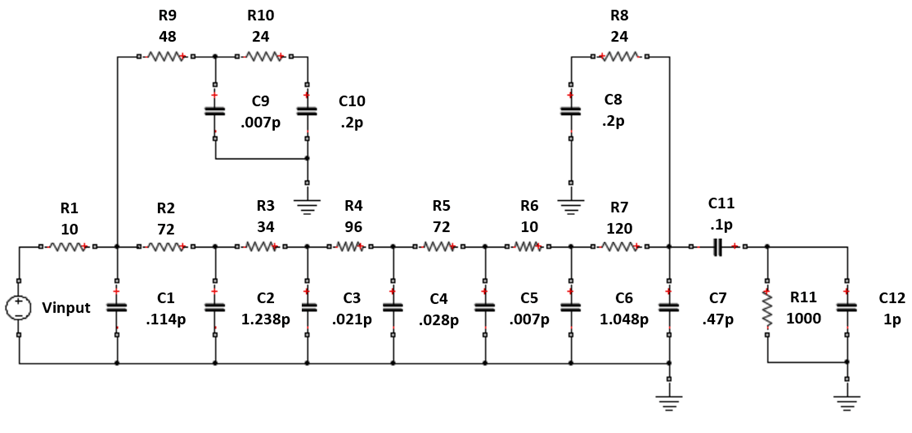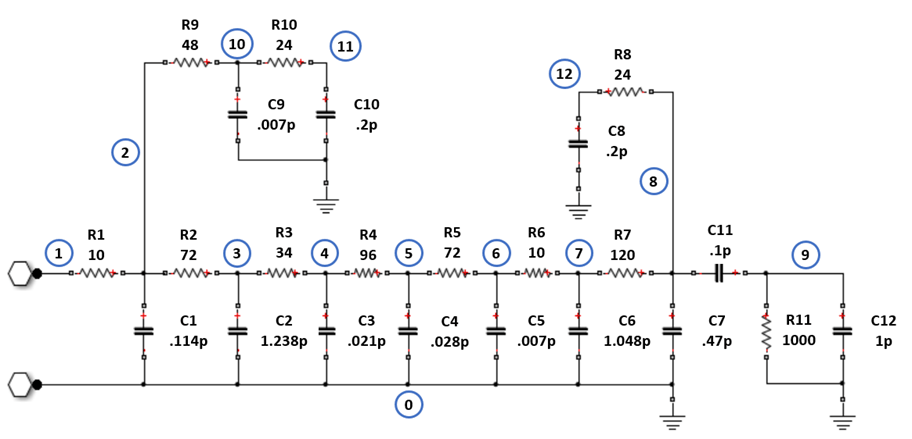# MOS Interconnect and Crosstalk

This example shows how to build and simulate an RC tree circuit using the RF Toolbox™.

In "Asymptotic Waveform Evaluation for Timing Analysis" (IEEE Transactions on Computer-Aided Design, Vol., 9, No. 4, April 1990), Pillage and Rohrer presented and simulated an RC tree circuit that models signal integrity and crosstalk in low- to mid-frequency MOS circuit interconnect. This example confirms their simulations using RF Toolbox software.

Their circuit, reproduced in the following figure, consists of 11 resistors and 12 capacitors. In the paper, Pillage and Rohrer:

• Apply a ramp voltage input

• Compute transient responses

• Plot the output voltages across two different capacitors, `C7` and `C12`.Figure 1: An RC tree model of MOS interconnect with crosstalk.

With RF Toolbox, you can programmatically construct this circuit in MATLAB and perform signal integrity simulations.

This example shows:

1. How to use `circuit`, `resistor`, and `capacitor` with the `add` function to programmatically construct the circuit.

2. How to use `clone`, `setports`, and `sparameters` objects to calculate S-parameters for each desired output over a wide frequency range.

3. How to use `s2tf` with `Zsource = 0` and `Zload = Inf` to compute the voltage transfer function from input to each desired output.

4. How to use `rationalfit` function to produce rational-function approximations that capture the ideal RC-circuit behavior to a very high degree of accuracy.

5. How to use `timeresp` function to compute the transient response to the input voltage waveform.

### Insert Node Numbers Into Circuit Diagram

Before building the circuit using `resistor` and `capacitor` objects, we must number the nodes of the circuit shown in figure 1.Figure 2: The circuit drawn with node numbers

### Programmatically Construct Circuit

Create a `circuit` and use the `add` function to populate the circuit with named `resistor` and `capacitor` objects.

```ckt = circuit('crosstalk'); add(ckt,[2 1],resistor(10,'R1')) add(ckt,[2 0],capacitor(0.114e-12,'C1')) add(ckt,[3 2],resistor(72,'R2')) add(ckt,[3 0],capacitor(1.238e-12,'C2')) add(ckt,[4 3],resistor(34,'R3')) add(ckt,[4 0],capacitor(0.021e-12,'C3')) add(ckt,[5 4],resistor(96,'R4')) add(ckt,[5 0],capacitor(0.028e-12,'C4')) add(ckt,[6 5],resistor(72,'R5')) add(ckt,[6 0],capacitor(0.007e-12,'C5')) add(ckt,[7 6],resistor(10,'R6')) add(ckt,[7 0],capacitor(1.048e-12,'C6')) add(ckt,[8 7],resistor(120,'R7')) add(ckt,[8 0],capacitor(0.47e-12,'C7')) add(ckt,[12 8],resistor(24,'R8')) add(ckt,[12 0],capacitor(0.2e-12,'C8')) add(ckt,[10 2],resistor(48,'R9')) add(ckt,[10 0],capacitor(0.007e-12,'C9')) add(ckt,[11 10],resistor(24,'R10')) add(ckt,[11 0],capacitor(0.2e-12,'C10')) add(ckt,[9 8],capacitor(0.1e-12,'C11')) add(ckt,[9 0],resistor(1000,'R11')) add(ckt,[9 0],capacitor(1e-12,'C12'))```

### Simulation Setup

The input signal used by Pillage and Rohrer is a voltage ramp from 0 to 5 volts with a rise time of one nanosecond and a duration of ten nanoseconds. The following MATLAB code models this signal with 1000 timepoints with a `sampleTime` of 0.01 nanoseconds.

The following MATLAB code also uses the `logspace` function to generate a vector of 101 logarithmically spaced analysis frequencies between 1 Hz and 100 GHz. Specifying a wide set of frequency points improves simulation accuracy.

```sampleTime = 1e-11; t = (0:1000)'*sampleTime; input = [(0:100)'*(5/100); (101:1000)'*0+5]; freq = logspace(0,11,101)';```

### Calculate S-Parameters For Each 2-Port Network

To calculate the response across both the `C7` and `C12` capacitors, two separate S-parameter calculations must be made: first, assuming the `C7` capacitor represents the output port, and second, assuming the `C12` capacitor represents the output port. To calculate the S-parameters for each setup:

1. Copy the original circuit `ckt` using the `clone` function.

2. Define the input and output ports of the network using the `setports` function.

3. Calculate the S-parameters using the `sparameters` object.

Calculate S-parameters with `C7` capacitor represents the output port.

```cktC7 = clone(ckt); setports(cktC7,[1 0],[8 0]) S_C7 = sparameters(cktC7,freq);```

Calculate S-parameters with `C12` capacitor represents the output port.

```cktC12 = clone(ckt); setports(cktC12,[1 0],[9 0]) S_C12 = sparameters(cktC12,freq);```

### Simulate Each 2-Port Network

To simulate each network:

1. The `s2tf` function, with `option = 2`, computes the gain from the source voltage to the output voltage. It allows arbitrary source and load impedances, in this case `Zsource = 0` and `Zload = Inf`. The resulting transfer functions `tfC7` and `tfC12` are frequency-dependent data vectors that can be fit with rational-function approximation.

2. The `rationalfit` function generates high-accuracy rational-function approximations. The resulting approximations match the networks to machine accuracy.

3. The `timeresp` function computes the analytic solution to the state-space equations defined by a rational-function approximation. This methodology is fast enough to enable one to push a million bits through a channel.

Simulate `cktC7` circuit.

```tfC7 = s2tf(S_C7,0,Inf,2); fitC7 = rationalfit(freq,tfC7); outputC7 = timeresp(fitC7,input,sampleTime);```

Simulate `cktC12` circuit.

```tfC12 = s2tf(S_C12,0,Inf,2); fitC12 = rationalfit(freq,tfC12); outputC12 = timeresp(fitC12,input,sampleTime);```

### Plot Transient Responses

The outputs match Figures 23 and 24 of the Pillage and Rohrer paper. Plot ramp response of low- to mid-frequency MOS circuit interconnect with crosstalk.

```figure plot(t,input,t,outputC7,'LineWidth',2) axis([0 2.5e-9 0 5.5]); title('Ramp Response of Low- to Mid-Frequency MOS Circuit Interconnect with Crosstalk'); xlabel('Time (sec)'); ylabel('Voltage (volts)'); legend('Vinput','V(C7)','Location','NorthWest');```Plot crosstalk in low- to mid-frequency MOS circuit interconnect with ramp input.

```figure plot(t,input,t,outputC12,'LineWidth',2) axis([0 5e-9 0 .5]) title('Crosstalk in Low- to Mid-Frequency MOS Circuit Interconnect with Ramp Input') xlabel('Time (sec)') ylabel('Voltage (volts)') legend('Vinput','V(C12)','Location','NorthEast')```### Verify Rational Fit Outside Fit Range

Though not shown in this example, you can also use the `freqresp` function to check the behavior of `rationalfit` function well outside the specified frequency range. The fit outside the specified range can sometimes cause surprising behavior, especially if frequency data near 0 Hz (DC) is not provided.

To perform this check for the rational-function approximation in this example, uncomment and run the following MATLAB code.

```% widerFreqs = logspace(0,12,1001); % respC7 = freqresp(fitC7,widerFreqs); % figure % loglog(freq,abs(tfC7),'+',widerFreqs,abs(respC7)) % respC12 = freqresp(fitC12,widerFreqs); % figure % loglog(freq,abs(tfC12),'+',widerFreqs,abs(respC12))```

For example on how to build and simulate this RC tree circuit using RFCKT objects, see MOS Interconnect and Crosstalk Using RFCKT Objects.